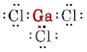# 7.3: Molecules with Lone Pairs

The VSEPR theory is able to explain and predict the shapes of molecules which contain lone pairs. In such a case the lone pairs as well as the bonding pairs are considered to repel and avoid each other. For example, since there are two bonds in the SnCl2 molecule, one might expect it to be linear like BeCl2. If we draw the Lewis diagram, though, we find a lone pair as well as two bonding pairs in the valence shell of the Sn atom:Ideally the three pairs of electrons should arrange themselves trigonally around the Sn atom, giving an angle of 120° between electron pairs, and hence between the two Cl atoms. Experimental measurements on SnCl2 reveal that the molecule is angular or V-shaped, as shown, but the Cl—Sn—Cl angle is significantly less than the predicted 120°. This smaller angle occurs because a lone pair of electrons is always “fatter” than a bonding pair. That is, a lone pair is like a bigger balloon which takes up more room and squeezes the bonding pairs closer together. This decreases the angle between bonding pairs in SnCl2, and hence between the bonded Cl atoms, from the expected value of 120°. The “fatness” of a lone pair compared with a bonding pair is shown in Fig. $$\PageIndex{1}$$ .

A lone pair also affects the structure of ammonia, NH3. Since this molecule obeys the octet rule, the N atom is surrounded by four electron pairs:If these pairs were all equivalent, we would expect the angle between them to be the regular tetrahedral angle of 109.5°. Experimentally, the angle is found to he somewhat less, namely, 107°. Again this is because the lone pair is “fatter” than the bonding pairs and able to squeeze them closer together.Figure $$\PageIndex{1}$$: Comparison of the electron clouds of a lone pair and a bonding pair. (a) The lone pair of electrons on the nitrogen in an ammonia molecule. (b) One of the three bonding pairs of electrons in the ammonia molecule. Boundary lines which enclose equal percentages of each electron cloud have been drawn. Note that the lone pair (a) takes up more space (is “fatter”) near the nitrogen nucleus than the bonding pair (b).

The electronic structure of the H2O molecule is similar to that of NH3 except that one bonding pair has been replaced by a lone pair:Here there are two “fat” lone pairs, and so the bonding pairs are squeezed even closer together than in NH3. The H―O―H angle is found to be 104.5°. The structures of BeCl2, BCl3, SnCl2, CCl4, NH3 and H2O include all the combinations of lone pairs and bonding pairs and all molecular shapes which are possible for two, three, and four pairs of electrons. These shapes, together with details of their geometries, are summarized in Fig. $$\PageIndex{2}$$ . Again, because of their frequent occurrence, it is wise to commit these to memory. Note in particular that the shape of a molecule is described in terms of the geometry of the nuclei and not of the electron clouds. For example, the shape of the NH3 molecule is described as a trigonal pyramid since the N nucleus forms the apex of a pyramid, slightly above an equilateral triangle of H nuclei. Although the electron-pair clouds are arranged in an approximate tetrahedron around the N nucleus, it is incorrect to describe the molecular shape as tetrahedral. The atomic nuclei are not at the corners of a tetrahedron.

Example $$\PageIndex{1}$$ : Molecular Geometry

Sketch and describe the geometry of the following molecules: (a) GaCl3, (b) AsCl3, and (c) AsOCl3.

Solution

a) Since the element gallium belongs to group III, it has three valence electrons. The Lewis diagram for GaCl3 is thusSince there are three bonding pairs and no lone pairs around the Ga atom, we conclude that the three Cl atoms are arranged trigonally and that all four atoms are in the same plane.

b) Arsenic belongs to group V and therefore has five valence electrons. The Lewis structure for AsCl3 is thusSince a lone pair is present, the shape of this molecule is a trigonal pyramid, with the As nucleus a little above an equilateral triangle of Cl nuclei.

c) The Lewis diagram for AsOCl3 is similar to that of AsCl3.Figure $$\PageIndex{2}$$ : The arrangement of electron pairs and the shapes of molecules which contain lone pairs. Bonding pairs are indicated in color and have purposely been made very thin for diagrammatic effect. Lone pairs are indicated in gray. Note that the geometry of these molecules is described in terms of the nuclei and not of the electron pairs; it is described in terms of the ball-and-stick diagrams shown in the figure.

Since there are four bonding pairs, the molecule is tetrahedral. Sketches of each of these molecules areThe VSEPR theory can also be applied to molecules which contain five and six pairs of valence electrons, some of which are lone pairs. We have not included such species here because the majority of compounds fall into the categories we have described.